# Resistance, resistors in series and parallel combination |Class 10 electricity Notes

## 8. Factors affecting of resistances of a conductor

Electric resistance of a conductor (or a wire) depends on the following factors
1. Length of the conductor: -
From equation 5 we can see that resistance of a conductor is directly proportional to its length. So, when length of the wire is doubled, its resistance also gets doubled; and if length of the wire is halved its resistance also gets halved.
Thus a long wire has more resistance then a short wire.
2. Area of cross-section:-
Again form equation 5 we see that resistance of a conductor is inversely proportional to its area of cross-section. So, when the area of cross-section of a wire is doubled, its resistance gets halved; and if the area of cross-section of wire is halved then its resistance will get doubled.
Thus a thick wire has less resistance and a thin wire has more resistance.
3. 3. Nature of material of conductor:-
Electrical resistance of a conductor also depends on the nature of the material of which it is made. For example a copper wire has less resistance then a nichrome wire of same length and area of cross-section.
4. 4. Effect of temperature:-
It has been found that the resistance of all pure metals increases on raising the temperature and decreases on lowering the temperature.
Resistance of alloys like manganin, nichrome and constantan remains unaffected by temperature.

## 9. Resistance of a system of resistors

• We know that current through a conductor depends upon its resistance and potential difference across its ends.
• In various electrical instruments resistors are often used in various combinations and Ohm�s Law can be applied to combination of resistors to find the equivalent resistance of the combination.
• The resistances can be combined in two ways
1. In series
2. In parallel
To increase the resistance individual resistances are connected in series combination and to decrease the resistance individual resistances are connected in parallel combination.

#### 9(a) Resistors in Series

• When two or more resistances are connected end to end then they are said to be connected in series combination.
• Figure below shows a circuit diagram where two resistors are connected in series combination.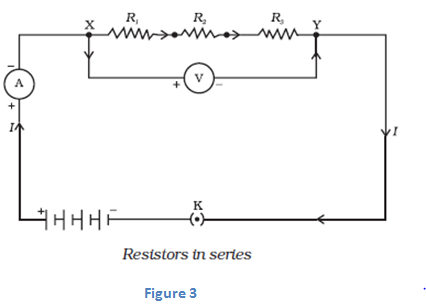• Now value of current in the ammeter is the same irrespective of its position in the circuit. So we conclude that " For a series combination of resistors the current is same in every part of the circuit or same current flows through each resistor "
• Again if we connect three voltmeters one across each resistor as shown below in the figure 4.The potential difference measured by voltmeter across each one of resistors R1 , R2 and R3 is V1 , V2 and V3 respectively and if we add all these potential differences then we get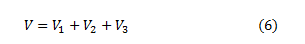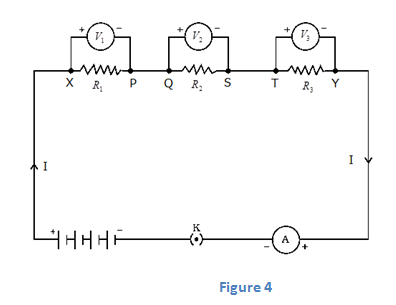This total potential difference V in equation 6 is measured to be equal to potential difference measured across points X and Y that is across all the three resistors in figure 3. So, we conclude that <"the total potential difference across a combination of resistors in series is equal to the sum of potential differences across the individual resistors."
• Again consider figure 4 where I is the current flowing through the circuit which is also the current through each resistor. If we replace three resistors joined in series by an equivalent single resistor of resistance R such that, the potential difference V across it, and the current I through the circuit remains same.
• Now applying Ohm�s law to entire circuit we get<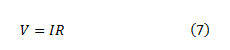On applying Ohm's law to the three resistors separately we have,<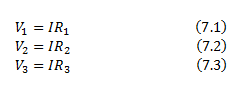From equation 6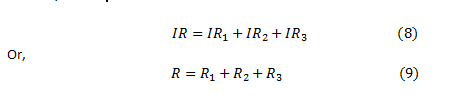So here from above equation 9 we conclude that when several resistances are connected in series combination, the equivalent resistance equals the sum of their individual resistances and is thus greater than any individual resistance.

#### 9(b) Resistors in parallel

• When two or more resistances are connected between the same two points they are said to be connected in parallel combination.
• Figure below shows a circuit diagram where two resistors are connected in parallel combination.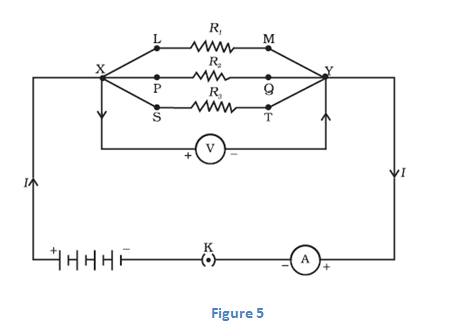#### IMPORTANT NOTE

1. When a number of resistors are connected in parallel, then the potential difference across each resistance is equal to the voltage of the battery applied.
2. When a number of resistances are connected in parallel, then the sum of the currents flowing through all the resistances is equal to total current flowing in the circuit.
3. When numbers of resistances are connected in parallel then their combined resistance is less than the smallest individual resistance. This happens because the same current gets additional paths to flow resulting decrease in overall resistance of the circuit
• To calculate the equivalent resistance of the circuit shown in figure 5 consider a battery B which is connected across parallel combination of resistors so as to maintain potential difference V across each resistor. Then total current in the circuit would be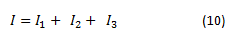Since potential difference across each resistors is V. Therefore, on applying Ohm's Law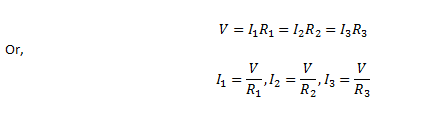Putting these values of current in equation 10 we have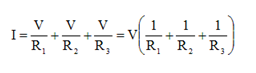If R is the equivalent resistance of parallel combination of three resistors heaving resistances R1, R2 and R3 then from Ohm's Law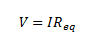Or,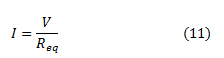Comparing equation (10) and (11) we get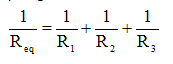• For resistors connected in parallel combination reciprocal of equivalent resistance is equal to the sum of reciprocal of individual resistances.
• Value of equivalent resistances for capacitors connected in parallel combination is always less than the value of the smallest resistance in circuit.

Question Calculate the electric current in the given circuit when

(a) Key K1 is open and K2 is closed

(b) both keys are closed

(c) Key K2 is open and K1 is closed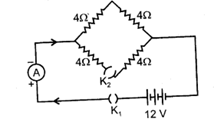Solution .

(a) When, key K1 is open and K2 is closed , then no current flows in the circuit as circuit is an open circuit.

(b) When both keys are closed a current begin to flow in the circuit. Let us consider the circuit given below where we have labelled above given circuit.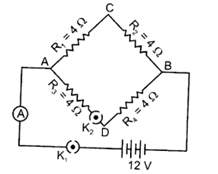How to determine equivalent resistance:-

(i) Now if you look closely at the circuit you would find that current is dividing at point A and combining again at point B.

(ii) Same amount of current (say I1) will flow through resistors R1 and R2 also same amount of current (say I2) will flow through resistors R3 and R4.

(iii)We are aware of the fact that “For a series combination of resistors the current is same in every part of the circuit or same current flows through each resistor”. So we can say that resistors R1 and R2 are connected in series combination. Similarly resistors R3 and R4 are also connected in series combination.

(iv) again as mentioned above in step (i) current is dividing at point A so we have different currents flowing through the combination of resistors R1 and R2 and resistors R3 and R4.

(v)So equivalent resistance of resistors R1 and R2 which is (R1 + R2) and equivalent resistance of resistors R3 and R4 which is (R3 + R4) are connected in parallel combination to each other as they have different amount of current flowing through them.

(vi)So, Equivalent resistance in the circuit would be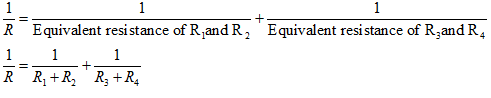Putting the values as given in the question we get

${\frac{1}{R} = \frac{1}{{4 + 4}} + \frac{1}{{4 + 4}} = \frac{1}{8} + \frac{1}{8} = \frac{1}{4}}$

So,

$R=4\Omega$

Electric current,

$I = \frac{V}{R} = \frac{{12}}{4} = 3A$

(c) When key K2 is open and K1 is closed, the part ADB will become an open circuit, So no current will flow in this part of the circuit.

Therefore, net resistance of the circuit will be

$R = {R_1} + {R_2} = 4 + 4 = 8\Omega$

Therefore, electric current.

$I = \frac{V}{R} = \frac{{12}}{8} = 1.5A$

Watch this tutorial for learning about how to solve resistance problems for class 10.

Given below are the links of some of the reference books for class 10 Science.

You can use above books for extra knowledge and practicing different questions.

### Practice Question

Question 1 Which among the following is not a base?
A) NaOH
B) $NH_4OH$
C) $C_2H_5OH$
D) KOH
Question 2 What is the minimum resistance which can be made using five resistors each of 1/2 Ohm?
A) 1/10 Ohm
B) 1/25 ohm
C) 10 ohm
D) 2 ohm
Question 3 Which of the following statement is incorrect? ?
A) For every hormone there is a gene
B) For production of every enzyme there is a gene
C) For every molecule of fat there is a gene
D) For every protein there is a gene

Note to our visitors :-

Thanks for visiting our website.